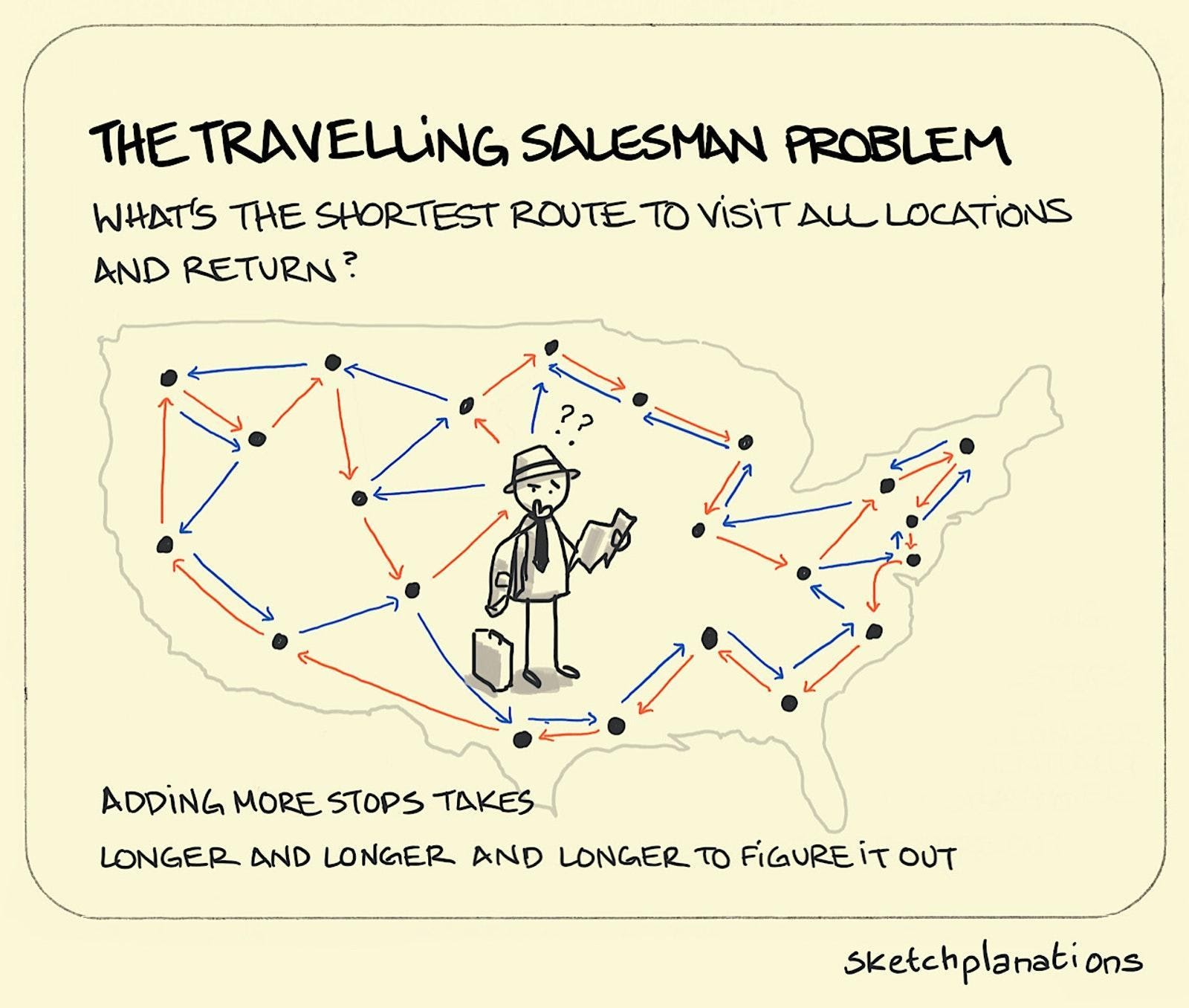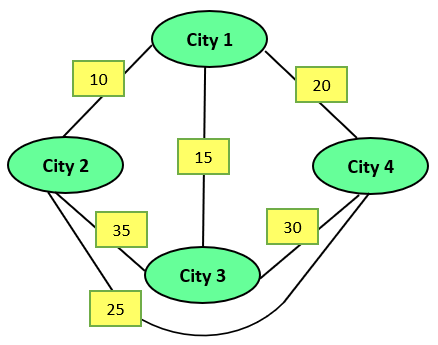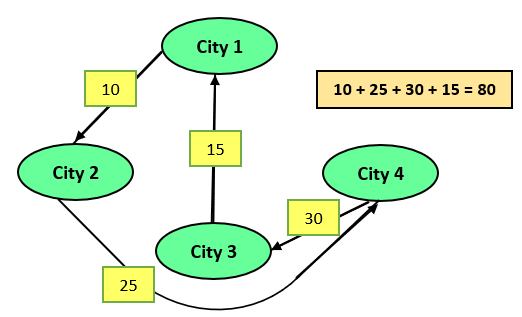# Travelling Salesman Problem | Part 1

## Introduction

The travelling salesman problem is one of the most searched optimisation problems. Many optimisation methods use the travelling salesman problem as a benchmark. Despite the problem's computational difficulty, various algorithms exist. These algorithms allow instances with tens of thousands of cities to be solved completely.

In this article, we’ll be looking at a basic version of this problem. We’ll understand the problem statement and various approaches to solve it.

So, Let’s get started!

## Problem Statement

The problem states that "What is the shortest possible route that helps the salesman visits each city precisely once and returns to the origin city, provided the distances between each pair of cities given?"Source: sketchplanations

Let’s understand this with the help of an example.

Example: Given, City1, City2, City3, City4 and distances between them as shown below. A salesman has to start from City 1, travel through all the cities and return to City1. There are various possible routes, but the problem is to find the shortest possible route.The various possible routes are:

Since we are travelling in a loop(source and destination are the same), paths like (1 - 2 - 3 - 4 - 1) and (1 - 4 - 3 - 2 - 1) are considered identical.

Out of the above three routes, route 2 is optimal. So, this will be the answer.Let’s understand the solution approach to this problem.

## Solution Approach(Brute force)

There are various ways to solve this problem. In this blog, we’ll discuss the brute force approach. The travelling salesman problem is a permutation problem. There can be n! total ways to solve permutation problems.

In this approach, we’ll generate all the possible permutations (routes). And find the one with the shortest distance.

The algorithm is as follows:

Step 1: Choose a city to act as a starting point(let’s say city1).

Step 2: Find all possible routes using permutations. For n cities, it will be (n-1)!.

Step 3: Calculate the cost of each route and return the one with the minimum cost.

Now, let’s move on to the implementation of the above algorithm. But, having known the algorithm to solve, we recommend you to give this problem a try by yourself on Codestudio.

## Implementation

The implementation is based on the example given above. We’ve passed the adjacent matrix of the graph given above. An adjacent matrix is a way to represent the graph.

### C++ Code

``````#include <bits/stdc++.h>
using namespace std;
#define V 4 // Total no of cities

{
// Store all cities apart from the source city in a vector.
vector<int> cities;
for (int i = 0; i < V; i++)
if (i != s)
cities.push_back(i);

int min_distance = INT_MAX;
do
{

// Taking starting Path distance as zero
int curr_distance = 0;

// compute current path distance
int k = s;
for (int i = 0; i < cities.size(); i++)
{
k = cities[i];
}

// update minimum
min_distance = min(min_distance, curr_distance);

}
//using following permutation method of C++
while(next_permutation(cities.begin(), cities.end()));

return min_distance;
}

int main()
{
//The adjacent matrix of the graph given in the example
{ { 0, 10, 15, 20 },
{ 10, 0, 35, 25 },
{ 15, 35, 0, 30 },
{ 20, 25, 30, 0 } };
//Starting city or source
int start = 0;
cout <<"The minimum cost is: "<<TSP_Implement(Adj_matrix, start) << endl;
return 0;
}``````

### Java Code

``````import java.util.*;
class Main{

static int V = 4;
static int travllingSalesmanProblem(int graph[][],
int s)
{
ArrayList<Integer> vertex = new ArrayList<Integer>();

for (int i = 0; i < V; i++)
if (i != s)

int min_path = Integer.MAX_VALUE;
do
{
int current_pathweight = 0;
int k = s;

for (int i = 0; i < vertex.size(); i++)
{
current_pathweight += graph[k][vertex.get(i)];
k = vertex.get(i);
}
current_pathweight += graph[k][s];
min_path = Math.min(min_path, current_pathweight);

} while (findNextPermutation(vertex));

return min_path;
}
public static ArrayList<Integer> swap(ArrayList<Integer> data,int left, int right){
int temp = data.get(left);
data.set(left, data.get(right));
data.set(right, temp);
return data;
}
public static ArrayList<Integer> reverse(ArrayList<Integer> data, int left, int right)
{
while (left < right)
{
int temp = data.get(left);
data.set(left++, data.get(right));
data.set(right--, temp);
}
return data;
}
public static boolean findNextPermutation(ArrayList<Integer> data)
{
if (data.size() <= 1)
return false;

int last = data.size() - 2;
while (last >= 0)
{
if (data.get(last) < data.get(last + 1))
{
break;
}
last--;
}
if (last < 0)
return false;

int nextGreater = data.size() - 1;
for (int i = data.size() - 1; i > last; i--)
{
if (data.get(i) > data.get(last))
{
nextGreater = i;
break;
}
}
data = swap(data,nextGreater, last);
data = reverse(data, last + 1, data.size() - 1);
return true;
}
public static void main(String args[])
{
int graph[][] = {{0, 10, 15, 20},
{10, 0, 35, 25},
{15, 35, 0, 30},
{20, 25, 30, 0}};
int s = 0;
System.out.println(travllingSalesmanProblem(graph, s));
}
}``````

### Python Code

``````from sys import maxsize
from itertools import permutations
V = 4

def travellingSalesmanProblem(graph, s):
vertex = []
for i in range(V):
if i != s:
vertex.append(i)

min_path = maxsize
next_permutation=permutations(vertex)
for i in next_permutation:
current_pathweight = 0
k = s
for j in i:
current_pathweight += graph[k][j]
k = j
current_pathweight += graph[k][s]
min_path = min(min_path, current_pathweight)

return min_path

if __name__ == "__main__":
graph = [[0, 10, 15, 20], [10, 0, 35, 25],
[15, 35, 0, 30], [20, 25, 30, 0]]
s = 0
print(travellingSalesmanProblem(graph, s))``````

### Output

``The minimum cost is: 80``

## Time and Space Complexity

• The time complexity of O(nn), where n is the number of cities.

Reason: The reason is that we’ve to check (n-1)! routes (i.e all permutations).

• The space complexity is O(n2)

Reason: The n*n adjacency matrix needs O(n2) space, and to store the remaining cities, we need O(n) space. The overall complexity will be O(n2).

This is not an optimal solution, so we'll explore some other approaches as well. Dynamic programming is one crucial algorithm. It is used when we can divide our problem into smaller subproblems.

The implementation of the travelling salesman problem using dynamic programming is explained in Part-2. So, go check it out!

1. What is TSP? Explain with example.
Answer: TSP is the travelling salesman problem consists of a salesperson and his travel to various cities. The salesperson must travel to each of the cities, beginning and ending in the same. The problem's challenge is that the travelling salesman wishes to minimise the trip's total length.

2. What type of problem is TSP?
Answer: TSP is an optimisation problem. In this, we have to optimise the route of travel

3. How is the travelling salesman problem related to the minimum spanning tree?
Answer: TSP and MST are two algorithmic problems that are closely connected. In particular, an open-loop TSP solution is a spanning tree, although it is not always the shortest spanning tree.

## Key Takeaways

In this article, we ran you through the travelling salesman problem. We saw a brute force approach to solve the problem.

There are various algorithmic paradigms such as Dynamic Programming and Backtracking to solve this problem. We’ll be seeing them in upcoming blogs. After reading this, it is recommended to move to the DP approach of TSP.

As we saw earlier, TSP is somewhat related to the minimum spanning tree. You can visit these two outstanding articles on the Prims and Kruskal's algorithm.